# Super US Coin Puzzle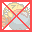Super US Coin Puzzle can also be played on mobile devices.Scan the QR code.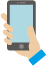## Rules

• Stack adjacent coins of the same amount.
• When the total amount of the stacked coins is the same as the coin more worth, the coins will be exchanged.
*If the total amount exceeds the amount of the coin more worth, the coins will not be exchanged.
•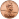x 5 = 5¢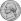•x 2 = 10¢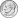• 10¢x 5 = 50¢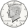• 50¢x 2 = \$1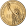• When you stack 5 \$1 coins, they will go into the piggy bank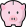automatically.
• The game is cleared when the specified number of \$1 coins are put in the piggy bank.
• To buy(remove) a sweet, merge it and the coins which is equal to or more worth than its price.
• The game is over when any coins cannot be moved or time is up.
Similar Games